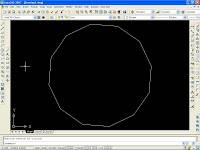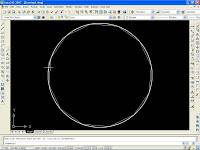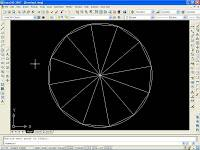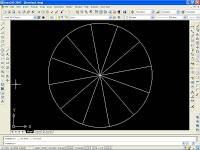# How Do I Divide A Circle Into 12 Equal Parts?

If you consider that every circle makes up 360 degrees (in terms of angles) from the center, then this comes easy. You need to divide the 360 degrees by 12, and you will be provided with 30 degrees. This means that every segment of the circle should consist of 30 degree angle segments. So all you need to do is use the protractor to give you the 30 degree angle, which will allow you to separate the separate parts of the circle.

You can't just do this by splitting the circle in half and then halving them until you finally reach the number of twelve. Not only will this involve a series of assumptions to even get to such a level of separation, but it will be generally messy and difficult to deal with. Using a ruler and a pencil simply won't do if you're looking at getting an accurate outline of a 12 part circle. Of course, if this is part of a mathematics question in academia then you're going to have to stick to the protractor method.

In an exam or during some kind of academic question you are being tested on your mathematical skills and your ability to use skills that have been taught to you to carry out tasks. Making an assumption and cutting up the circle with a ruler and a pencil does not demonstrate these skills and it does not demonstrate your mathematical ability. Instead it shows that you are unable to apply skills and that you do not take accuracy seriously within academia. This can be problematic and lose you marks in exams.

Stick to the method outlined that uses the protractor and of course the compass, and you will be sure that you will exceed in this area of mathematics and get an accurate result every time that you do this.
thanked the writer.
For any circle, measure the distance from the center of the circle to any point on the circle.
This is the radius of the circle. Choose a point on the circle, mark it. Starting from the point, use a ruler to find a point on the circle that is one radius away from the initial point. Mark it. Do this again starting at the second point. Repeat from the newest point until you get back to the first point. If you have measured correctly, you will arrive at exactly that point. Draw lines connecting each pair of consecutive points on the circle. Using your ruler, find the middle of each of these lines. Use your ruler to draw a line through each of these middle points, from the center of the circle to a point on the circle. These six points, along with the original six points, will divide the circle into 12 equal parts. Draw lines from the center to each of the 12 points. You will now have 12 sections of equal area. The arc of each of the 12 sections, will be equal in length to each of the other arcs.
thanked the writer.
You have a 21 inch circle that you need to divide into 12 parts

Do you mean 21 inch in diameter or 21 inch in radius. ??? Circle is measure usually in radius.

Every circle is 360 degrees angle from centre. Divide 360 by 12 and you get . 30.
That means each segment would be of 30 degrees. Now divide circle in 12 segments , each angle is 30 degrees.
thanked the writer.
Anonymous commented
Just look at a clock
Put Cross hairs on your circle. By doing this, you have divided the circle into 4 parts. 360 degrees is a full circle. 360 divided by 12 = 30. Start at the "top" of your circle and mark 30 degrees. This will occur 3 times in each 1/4 segment of your circle. 3 X 4 = 12. You can place numbers into each 30 degree section, starting with 1 in the upper right sector...this will make a clock.
thanked the writer.
1. Draw a circle with the desired radius (R).
2. Starting at arbitrary point A, and using the same radius, mark off points around the circle that are R apart. You have divided the circle into 6 equal parts.
3. Pick one of those points adjacent to A and call it B.
4. From points A and B, draw arcs of radius R that intersect outside the circle. Call the intersection point J.
5. Draw a line from point J to the center of the circle. It will cross the circle exactly halfway between points A and B. Call that intersection point K.
6. Mark off the circle at intervals of R, beginning at point K. You have now added 6 points halfway between the 6 points you marked in step 2. Your circle is now divided into 12 equal parts.

thanked the writer.
Hoe do I divide a &; and 7" circle into 12 equal parts
thanked the writer.

Here is how you can divide your circle to get equally for each parts with Autocad.

1.Firstly draw a polygon for example with 12 corners.2. Then draw a circle corresponded to the center of polygon .3.Draw line from polygon edge to divide the circle equally .4.Lastly, delete the polygon object and now you have your circle that equally divided into 12 partsthanked the writer.
The simplest way is
Just draw horizontal and vertical diameters to the circle. Now, divide each diameter into 4 equal parts and draw lines to form a grid. You can see now when the lines intersect the circle you see 12 points. Hurray there you are just joint all the points on the circle to their opposite points.
thanked the writer.
Look at it like a clock and go from there.
thanked the writer.